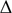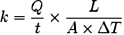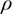# Material Properties Definitions

Coefficient of Thermal Expansion – When solids or liquids are heated or cooled, they expand or contract due to stored energy and the length of molecular bonds. This response of a material to a change in temperature is expressed as the material’s coefficient of thermal expansion.

The coefficient of thermal expansion is used in two ways:

• as a volumetric thermal expansion coefficient
• as a linear thermal expansion coefficient

These characteristics are closely related. The volumetric thermal expansion coefficient can be measured for all substances of condensed matter (liquids and solid state). The linear thermal expansion can only be measured in the solid state and is common in engineering applications.

When thin layers of two materials with rather different CTEs are bound together and then heated or cooled, stress builds resulting in bending, cracking or delamination.

Glass transition temperature – A material’s Tg, is the temperature below which molecules have little relative mobility. Tg is usually applicable to wholly or partially amorphous phases such as glasses and plastics

Above Tg, the secondary, non-covalent bonds between the polymer chains become weak in comparison to thermal motion, and the polymer becomes rubbery and capable of elastic or plastic deformation without fracture. This behavior is one of the things which make most plastics useful. But such behavior is not exhibited by crosslinked thermosetting plastics which, once cured, are set for life and will shatter rather than deform, never becoming plastic again when heated, nor melting.

Thermal conductivity – Thermal conductivity, k, is the property of a material that indicates its ability to conduct heat.

It is defined as the quantity of heat, Q, transmitted in time t through a thickness L, in a direction normal to a surface of area A, due to a temperature differenceT, under steady state conditions and when the heat transfer is dependent only on the temperature gradient.

thermal conductivity = heat flow rate x distance / (area x temperature difference)Dielectric constant – Dielectrics are usually insulators. Examples include ceramic, mica, glass, plastics, and the oxides of various metals. Some liquids and gases can serve as good dielectric materials. Dry air is an excellent dielectric, and is used in variable capacitors and some types of transmission lines. Distilled water is a good dielectric if kept free from impurities and has a relative dielectric constant of about 80. Vacuum is 1 by definition. Air is 1.0005.

Young’s modulus or Tensile modulus – Young’s modulus is also known as the modulus of elasticity, elastic modulus or tensile modulus. It is a measure of the stiffness of a given material. It is defined as the limit, for small strains, of the rate of change of stress with strain. This can be experimentally determined from the slope of a stress-strain curve created during tensile tests conducted on a sample of the material. It is named after Thomas Young an English physicist.

Tensile strength – Tensile strength measures the force required to pull something such as rope, wire, or a structural beam to the point where it breaks. Specifically, the tensile strength of a material is the maximum amount of tensile stress that it can be subjected to before failure. The definition of failure can vary according to material type and design methodology
There are three typical definitions of tensile strength:

• Yield strength – The stress a material can withstand without permanent deformation.
• Ultimate strength – The maximum stress a material can withstand.
• Breaking strength – The stress coordinate on the stress-strain curve at the point of rupture.

Breakdown voltage – Breakdown Voltage (Insulator) is the minimum voltage that makes an insulator react as a conductor. It is a parameter of an insulator that defines the maximum voltage difference that can be applied across the material before the insulator collapses and conducts. This may create a weak point in the insulator from a molecular change created by the current flow.

Density – Density (symbol:– Greek: rho) is a measure of mass per unit of volume. The higher an object’s density, the higher its mass per volume. The average density of an object equals its total mass divided by its total volume.

Typical values: Water = 1; Al=2.7; Cu=8.9; Pb=11.3; Hg=13.6; W=19.3; Au=19.3; Os=22.6

Specific heat capacity – The specific heat capacity (c or s, is also called specific heat or SHC) of a substance is defined as heat capacity per unit mass. It is the amount of energy required to raise the temperature of one kilogram of the substance by one kelvin. Heat capacity can be measured by using calorimetry.

It is the amount of energy (measured in ergs) required to raise the temperature of one gram of the substance by one degree Celsius (erg/g·°C). Other units of specific heat capacity include calories per gram degree Celsius (cal./g·°C) or cal. /(g·°K) and Btu per pound degree Fahrenheit (Btu/lb.·°F).

The symbols cp and cv are often used to denote specific heat capacity at constant pressure and at constant volume.
Substances with low specific heat such as metals require less input energy to increase their temperature. Substances with high specific heat such as water require much more energy to increase their temperature. The specific heat can also be interpreted as a measure of how well a substance preserves its temperature, i.e. “stores” heat, hence the term “heat capacity”.